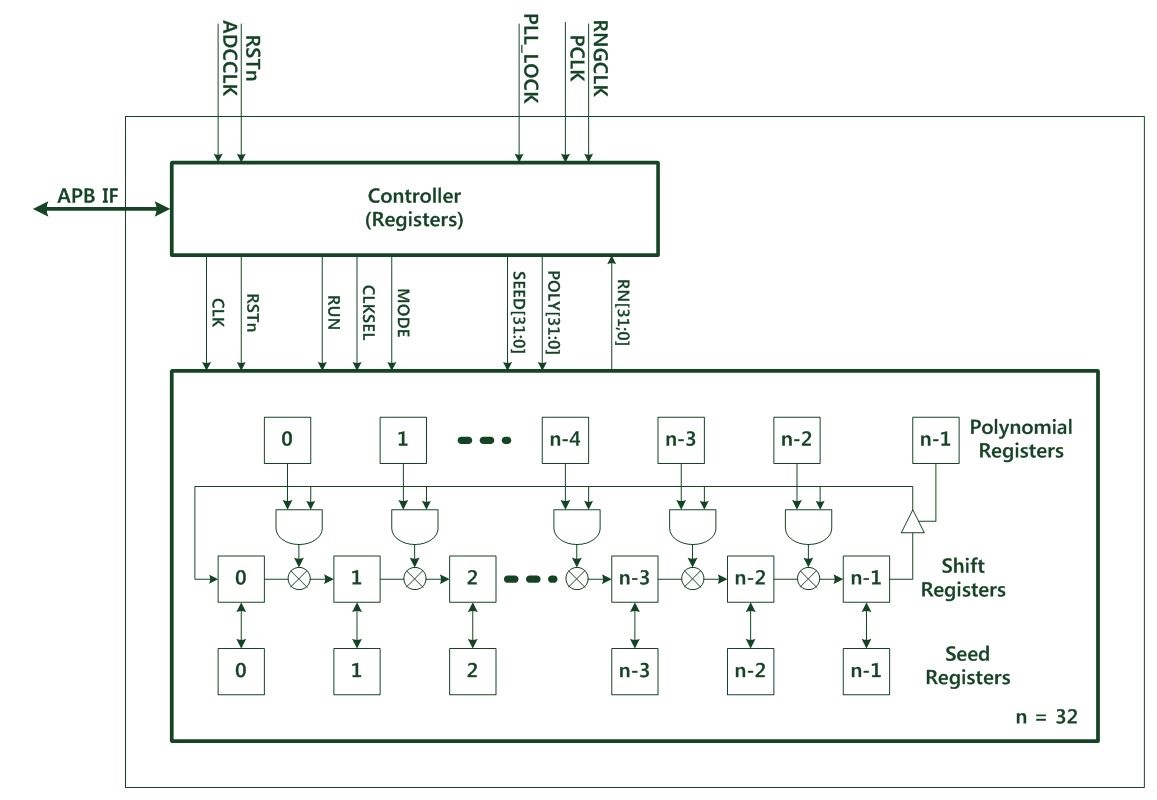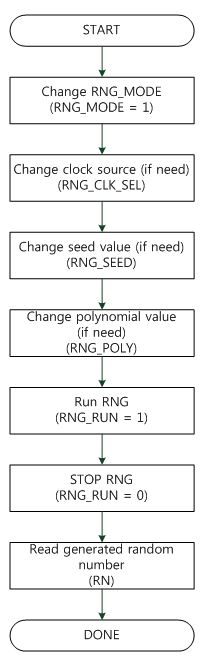# Random number generator (RNG)

## Introduction​

RNG is a 32bit random number generator. RNG generates power on random number when power on reset. RNG can run/stop by software. RNG seed value and polynomial of RNG can be modified by software.

## Features​

• 32bit pseudo random number generator
• Formula of pseudo random number generator (polynomial) can be modified.
• Seed value of random generator can be modified.
• Support power on reset random value
• Random value can be obtained by control start/stop by software.

## Functional description.​

The below Figure shows the RNG block diagram.### Operation-RNG​

A random number is automatically generated after powering on reset, Follow the procedure below to manually generate a random number.

1. Change MODE to start/stop by register.
2. Change clock source/seed value/polynomial value if need.
3. Run and Stop the RNG.